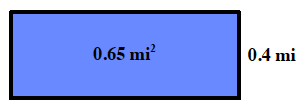### Home > CC1MN > Chapter 8 > Lesson 8.2.5 > Problem8-118

8-118.

Molly is raising cows. She has a pasture for them that is $0.65$ square miles in area. One dimension of the pasture is $0.4$ miles long.

1. What is the length of the other side of the pasture?

Remember the formula to find area:
Area = base · height

Substitute the values into the equation and solve for the missing variable.

$0.65 \text{ mi}^2 = (\text{base})(0.4 \text{ mi})$The other side of the pasture is $1.625$ miles long.

2. How much fencing will she need to go around the pasture?

Now that you know the lengths of all $4$ sides of the pasture, find the perimeter.